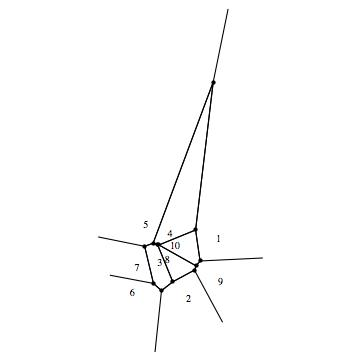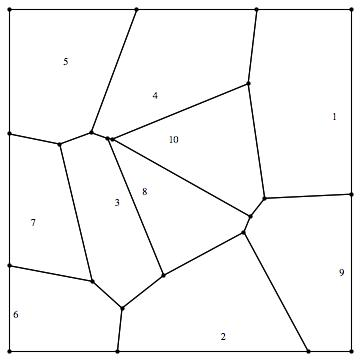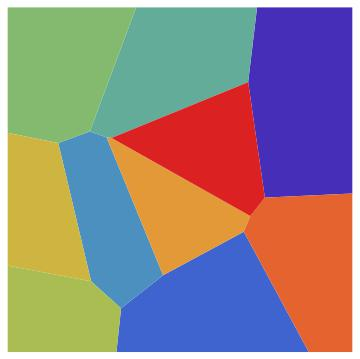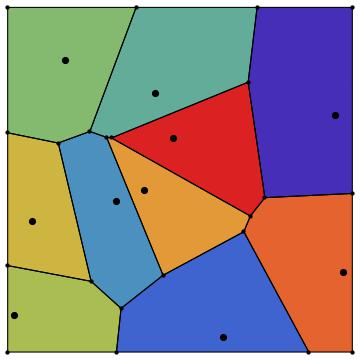# Voronoi Diagrams in Mathematica

Ecological models sometimes find very unexpected applications. Work on wolf territory modeling by Mark Lewis’s research group at the University of Alberta has been employed by researchers studying gang territories in Los Angeles. You can check out that paper by Smith, Bertozzi, Tita, and Valasik in the September 2012 issue of the Journal of Discrete and Continuous Dynamical Systems.

This Smith et al. paper uses Voronoi diagrams as a null model for territorial use. Voronoi diagrams pop up in modeling forest canopy structure, and a variety of other ecological applications. Given the their utility, I am devoting today’s post to an overview of computing and displaying them in Mathematica. First, a brief layperson’s description of a Voronoi diagram. Start with a set of reference points in space. A Voronoi diagram associates a cell (a region of space) to each reference point. A reference point’s cell contains all of the points that are closer to that reference point than any other reference point.

For a concrete example, consider the locations of Burger King restaurants in Omaha. You can partition a map of Omaha into cells, where each cell contains exactly one Burger King. The cell of Burger King #1 contains all the parts of Omaha that are closer to Burger King #1 than they are to any other Burger King.

If you are a mathy type, you’ll recall that the dual graph of a Voronoi diagram for a set of reference points is the Delaunay triangulation of that set of points.

Voronoi diagrams can involve non-standard metrics, but we’ll stick with vanilla Voronoi diagrams in this example.

Given a set of points, it’d be nice to have a computer calculate and display the associated Voronoi diagram for you. This can be accomplished easily In Mathematica.

You can follow along with the code below.

First, be sure to load the computational geometry package:

`` << ComputationalGeometry```

Next, generate a set of reference points:

``````refpoints=Table[ {RandomVariate[UniformDistribution[{0,1}]],
RandomVariate[UniformDistribution[{0,1}]]},
{10} ]``````

Next, plot the Voronoi diagram using the command `DiagramPlot[]`:

``DiagramPlot[refpoints]``Let’s dig a little deeper. How does Mathematica represent the data in the above plot? Try typing:

``VoronoiDiagram[refpoints]``

You get a list of two lists, of the general form `{coords, polys}`. The first list, which we will call coords, is a list of the points of the vertices in the Voronoi diagram. Each entry in the second list, which we will call polys, specifies the polygon that surrounds a given reference point. An entry in polys typically looks like `{1,{12,9,11,14,13}}`. This means that the polygon associated with the first reference point (because of the `1`), is found by starting at the 12th point of coords, drawing a line to the 9th point of coords, drawing a line to the 11th point of coords, etc.

You’ll also notice that some points of coords are not points at all, but rather rays. This is because we are dividing the plane into cells, and hence some cells are unbounded.

What if you wanted to construct a Voronoi diagram, but restrict it to a region, say the unit square?

Here we use the command `BoundedDiagram[]`. Try typing:

``BoundedDiagram[ { {0,0},{1,0},{1,1},{0,1} }, refpoints ]``

This gives us an output much like the VoronoiDiagram command, but now restricted to the unit square. The first argument of `BoundedDiagram` is the polygon that you are restricting your space to; in the case of the unit square, this polygon is described by listing the vertices in counterclockwise fashion.

Let’s see what that looks like. Try typing:

``````{coords, polys}= BoundedDiagram[ { {0,0},{1,0},{1,1},{0,0} },
refpoints];
DiagramPlot[refpoints, coords, polys]``````Don’t like the pesky number labels for the reference points? Prefer dots? Type:

``````Show[ DiagramPlot[ refpoints, coords, polys, LabelPoints-> False],
ListPlot[refpoints, AspectRatio->1, PlotRange->{{0,1},{0,1}},
PlotStyle->{PointSize[0.02],Red} ] ]``````Ok! Now let’s color in our regions and make a pretty picture. There should be an easy option for that in `DiagramPlot[]`, right? Alas, no.

Instead, you will have to use coords and polys to build the graphics primitives. First define a set of colors. For example, try typing:

``colors=Table[ColorData[“Rainbow”][k/10],{k,1,10}];``

If you are new to Mathematica, note that `&` is the notation for a pure function, and `/@` is the symbol for mapping a function to a list.

Now, build the actual polygons. This is adapted from a nice piece of code from Takashi Yoshino at Wakayayama University.

``````Transpose[{colors,
Polygon[#] & /@ (coords[[#]] & /@ (#[] & /@ polys))}]// Graphics``````And, with references points, colors, and boundary lines all together:

``````Show[Transpose[{colors,
Polygon[#] & /@ (coords[[#]] & /@ (#[] & /@ polys))}] // Graphics,
DiagramPlot[refpoints,coords, polys, LabelPoints -> False],
ListPlot[refpoints, AspectRatio -> 1,
PlotRange -> {{0, 1}, {0, 1}},
PlotStyle -> {PointSize[0.02], Black}]]``````